GATE  >  Mining Engineering - (MN) 2018 2018 GATE Paper (Practice Test)

# Mining Engineering - (MN) 2018 2018 GATE Paper (Practice Test) - GATE

Test Description

## 65 Questions MCQ Test GATE Past Year Papers for Practice (All Branches) - Mining Engineering - (MN) 2018 2018 GATE Paper (Practice Test)

Mining Engineering - (MN) 2018 2018 GATE Paper (Practice Test) for GATE 2023 is part of GATE Past Year Papers for Practice (All Branches) preparation. The Mining Engineering - (MN) 2018 2018 GATE Paper (Practice Test) questions and answers have been prepared according to the GATE exam syllabus.The Mining Engineering - (MN) 2018 2018 GATE Paper (Practice Test) MCQs are made for GATE 2023 Exam. Find important definitions, questions, notes, meanings, examples, exercises, MCQs and online tests for Mining Engineering - (MN) 2018 2018 GATE Paper (Practice Test) below.
Solutions of Mining Engineering - (MN) 2018 2018 GATE Paper (Practice Test) questions in English are available as part of our GATE Past Year Papers for Practice (All Branches) for GATE & Mining Engineering - (MN) 2018 2018 GATE Paper (Practice Test) solutions in Hindi for GATE Past Year Papers for Practice (All Branches) course. Download more important topics, notes, lectures and mock test series for GATE Exam by signing up for free. Attempt Mining Engineering - (MN) 2018 2018 GATE Paper (Practice Test) | 65 questions in 180 minutes | Mock test for GATE preparation | Free important questions MCQ to study GATE Past Year Papers for Practice (All Branches) for GATE Exam | Download free PDF with solutions
 1 Crore+ students have signed up on EduRev. Have you?
Mining Engineering - (MN) 2018 2018 GATE Paper (Practice Test) - Question 1

### “From where are they bringing their books? ________ bringing _______ books from _____.”  The words that best fill the blanks in the above sentence are

Mining Engineering - (MN) 2018 2018 GATE Paper (Practice Test) - Question 2

### “A _________ investigation can sometimes yield new facts, but typically organized ones are more successful.”   The word that best fills the blank in the above sentence is

Mining Engineering - (MN) 2018 2018 GATE Paper (Practice Test) - Question 3

### The area of a square is ��. What is the area of the circle which has the diagonal of the square as its diameter?

Mining Engineering - (MN) 2018 2018 GATE Paper (Practice Test) - Question 4

What would be the smallest natural number which when divided either by 20 or by 42 or by 76 leaves a remainder of 7 in each case?

Mining Engineering - (MN) 2018 2018 GATE Paper (Practice Test) - Question 5

What is the missing number in the following sequence?

2,  12,  60,  240,  720,  1440,  _____,  0

Mining Engineering - (MN) 2018 2018 GATE Paper (Practice Test) - Question 6

In appreciation of the social improvements completed in a town, a wealthy philanthropist decided to gift Rs 750 to each male senior citizen in the town and Rs 1000 to each female senior citizen. Altogether, there were 300 senior citizens eligible for this gift. However, only 8/9th of the eligible men and 2/3rd of the eligible women claimed the gift. How much money (in Rupees) did the philanthropist give away in total?

Mining Engineering - (MN) 2018 2018 GATE Paper (Practice Test) - Question 7

If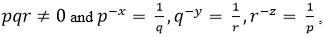what is the value of the product XYZ?

Mining Engineering - (MN) 2018 2018 GATE Paper (Practice Test) - Question 8

In a party, 60% of the invited guests are male and 40% are female. If 80% of the invited guests attended the party and if all the invited female guests attended, what would be the ratio of males to females among the attendees in the party?

Mining Engineering - (MN) 2018 2018 GATE Paper (Practice Test) - Question 9

In the figure below, ∠DEC + ∠BFC is equal to ____________ .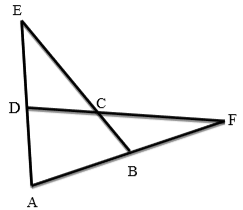Mining Engineering - (MN) 2018 2018 GATE Paper (Practice Test) - Question 10

A six sided unbiased die with four green faces and two red faces is rolled seven times. Which of the following combinations is the most likely outcome of the experiment?

Mining Engineering - (MN) 2018 2018 GATE Paper (Practice Test) - Question 11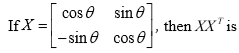Mining Engineering - (MN) 2018 2018 GATE Paper (Practice Test) - Question 12

The values of x satisfying the following condition are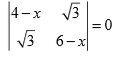Mining Engineering - (MN) 2018 2018 GATE Paper (Practice Test) - Question 13

An azimuth of 330° corresponds to a quadrant bearing of

Mining Engineering - (MN) 2018 2018 GATE Paper (Practice Test) - Question 14

Tri-cone drill bit is a type of

Mining Engineering - (MN) 2018 2018 GATE Paper (Practice Test) - Question 15

Exposure of weak roof in junctions of a development district in a coal mine can be decreased by

Mining Engineering - (MN) 2018 2018 GATE Paper (Practice Test) - Question 16

The property that CANNOT be determined from uniaxial compressive strength test of a rock sample fitted with strain gauges is

Mining Engineering - (MN) 2018 2018 GATE Paper (Practice Test) - Question 17

The radial stress concentration around a long circular tunnel excavated in rock is given by the curve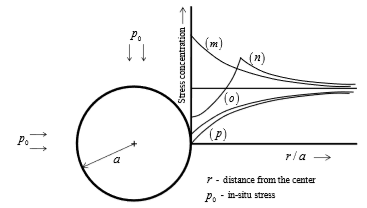Mining Engineering - (MN) 2018 2018 GATE Paper (Practice Test) - Question 18

Ward-Leonard system is provided in the winding system in order to restrict

Mining Engineering - (MN) 2018 2018 GATE Paper (Practice Test) - Question 19

The equipment NOT related to extraction of coal from longwall face operation is

Mining Engineering - (MN) 2018 2018 GATE Paper (Practice Test) - Question 20

The correct figure depicting the extraction of contiguous seams in bord and pillar working is indicated by

Mining Engineering - (MN) 2018 2018 GATE Paper (Practice Test) - Question 21

The significance of ‘potentially explosive mixture’ in Coward flammability diagram is -

Mining Engineering - (MN) 2018 2018 GATE Paper (Practice Test) - Question 22

Considering ‘I’ as ‘intake’ and ‘R’ as ‘return’, the ventilation symbol for the shaft-bottom air-lock in a coal mine is

Mining Engineering - (MN) 2018 2018 GATE Paper (Practice Test) - Question 23

From a coal seam of a mine 1000 tonnes of coal is produced per day. The seam has inflammable gas emission rate of 14000 m3 per day. Percentage of inflammable gas in general body of air is 0.14. The gassiness of the seam is

Mining Engineering - (MN) 2018 2018 GATE Paper (Practice Test) - Question 24

The temperature profiles and the plume patterns that are most likely to result are given in the figures. The dotted line represents ‘adiabatic lapse rate’ and the bold line represents ‘environmental lapse rate’. The WRONG combination is

Mining Engineering - (MN) 2018 2018 GATE Paper (Practice Test) - Question 25

The inventory pattern shown does NOT represent the following.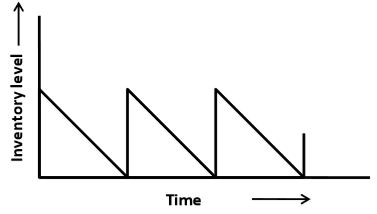Mining Engineering - (MN) 2018 2018 GATE Paper (Practice Test) - Question 26

The figure depicts a transportation problem along with the solution. The correct statement is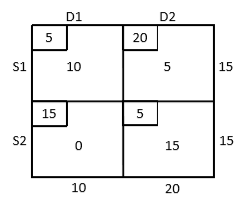Mining Engineering - (MN) 2018 2018 GATE Paper (Practice Test) - Question 27

The degree of the differential equation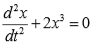is ________________-.

Mining Engineering - (MN) 2018 2018 GATE Paper (Practice Test) - Question 28

The slope of the line connecting the points (20, 6) and (40, 8) is ______________.

Mining Engineering - (MN) 2018 2018 GATE Paper (Practice Test) - Question 29

Two contours of RL 60 m and 70 m are separated by 34 m measured along dip of the seam. The dip of the seam in degree is ________________.

Mining Engineering - (MN) 2018 2018 GATE Paper (Practice Test) - Question 30

The RL of the initial station is 200 m. If ∑ BS = 1.54 m and ∑ FS = 0.45 m , then the RL of the last station in m is ________________.

Mining Engineering - (MN) 2018 2018 GATE Paper (Practice Test) - Question 31

For the conveyor belt drive shown, the tension on the tight side (T1 ) is double that of the slack side. The coefficient of friction between belt and drum is 0.21. The minimum angle of lap (in degree) to avoid slippage of the belt is ________________.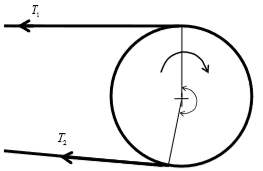Mining Engineering - (MN) 2018 2018 GATE Paper (Practice Test) - Question 32

In a bord and pillar development district, 6 headings driven along the strike direction are surrounded by panel barriers on the dip and rise sides. The maximum possible number of faces for the panel is ___________________.

Mining Engineering - (MN) 2018 2018 GATE Paper (Practice Test) - Question 33

A roadway in a mine, a single light source as shown, emits light uniformly in all directions. The floor level illumination at station A is 20 lux. The floor level illumination at a point B in lux is ______________.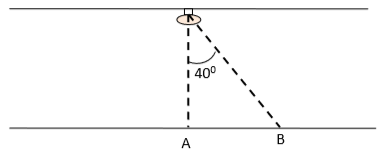Mining Engineering - (MN) 2018 2018 GATE Paper (Practice Test) - Question 34

A system of two identical components connected in series has reliability of 0.25. The reliability of each component is _______________.

Mining Engineering - (MN) 2018 2018 GATE Paper (Practice Test) - Question 35

The operational status of an HEMM in a shift is shown in the diagram. The availability of the machine in % is _____________________.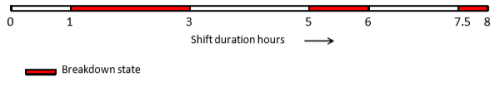Mining Engineering - (MN) 2018 2018 GATE Paper (Practice Test) - Question 36

If c is a constant, the solution of the differential equation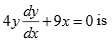is

Mining Engineering - (MN) 2018 2018 GATE Paper (Practice Test) - Question 37

Match the following blasting elements with the corresponding initiators.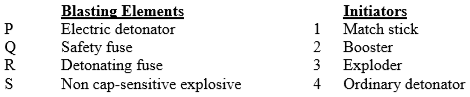Mining Engineering - (MN) 2018 2018 GATE Paper (Practice Test) - Question 38

The following plot is developed for a rock type after a series of triaxial tests. The uniaxial compressive strength and tensile strength of the rock type, respectively, in MPa, are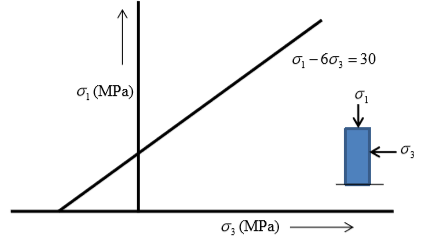Mining Engineering - (MN) 2018 2018 GATE Paper (Practice Test) - Question 39

Match the following in the context of environmental management.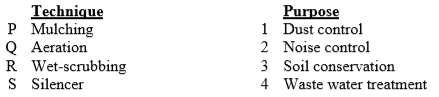Mining Engineering - (MN) 2018 2018 GATE Paper (Practice Test) - Question 40

A panel in coal mine produces 400 tonne per day. The number of persons employed in each of the three shifts is 110, 130 and 120. As per CMR, the minimum quantity of air that needs to be circulated at the last ventilation connection of the panel in m3/min is

Mining Engineering - (MN) 2018 2018 GATE Paper (Practice Test) - Question 41

A right conical iron ore stack on level ground of height 10 m has 60% Fe. The height of the conical stack is extended up to 20 m using iron ore of 50% Fe. The angle of repose of iron ore is 38o. The mean grade of the final stack in % Fe is ___________________.

Mining Engineering - (MN) 2018 2018 GATE Paper (Practice Test) - Question 42

The feasible region of a linear programming problem is shown in the figure. The maximum value of the objective function Z = 4 X+3Y is ____________________.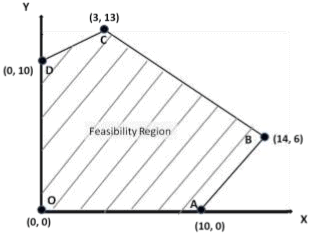Mining Engineering - (MN) 2018 2018 GATE Paper (Practice Test) - Question 43

Cash flow of a project of duration 4 years is shown. The uniform income ‘R’, in Rs. crores, at the end of 2nd, 3rd and 4th years for an internal rate of return of 10% is ______________.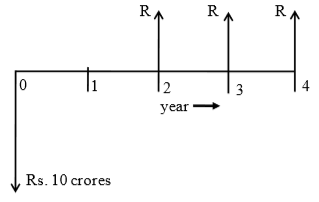Mining Engineering - (MN) 2018 2018 GATE Paper (Practice Test) - Question 44

The grade values of alumina at three sample locations (A, B and C) in a bauxite deposit are as shown. Using the ‘inverse distance’ method, the computed grade in % alumina at location ‘Z’ is ___________________.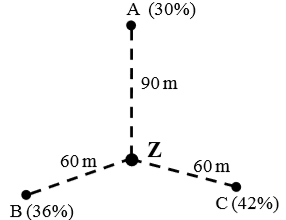Mining Engineering - (MN) 2018 2018 GATE Paper (Practice Test) - Question 45

Economic feasibility of two methods is examined for meeting a targeted mine production. On a yearly basis, the following cost parameters (Rs in crores) are applicable for these methods.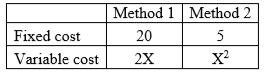The annual rate of production, ‘X’ in million tonnes for which both the methods will yield the same operating cost is ___________________.

Mining Engineering - (MN) 2018 2018 GATE Paper (Practice Test) - Question 46

A person standing 50 m away from an HEMM experiences 90 dB sound pressure level. If the person moves to a new location that is 70 m away from the HEMM, the sound pressure level experienced by the person, in dB, becomes _________________.

Mining Engineering - (MN) 2018 2018 GATE Paper (Practice Test) - Question 47

A portion of a ventilation system has two splits as shown. Split ‘A’ has a resistance of 0.2 Ns2/m8 and a regulator of size 2.0 m2. The resistance of split ‘B’ in Ns2/m8 is ________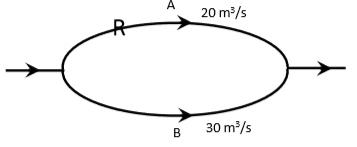Mining Engineering - (MN) 2018 2018 GATE Paper (Practice Test) - Question 48

Air enters a bord and pillar panel at 10 ppm CO and 20.78% O2. The air at the panel return has 80 ppm CO and 20.52% O2. The ‘Graham’s ratio’ for the status of fire in the panel in % is __________________.

Mining Engineering - (MN) 2018 2018 GATE Paper (Practice Test) - Question 49

In a sublevel stope, 20 m high excavation is made with ring drilling in a 6 m wide orebody.  In an effective shift time of 6 hrs, three rings are blasted resulting in 9 m extraction length. LHDs of 5.0 m3 bucket capacity and cycle time of 5 minutes are used for ore transportation. The number of LHDs required for this operation is _________________.

Mining Engineering - (MN) 2018 2018 GATE Paper (Practice Test) - Question 50

A coal seam lying at a depth of 200 m is developed by bord and pillar method. Pillars are 30 m centre to centre with a gallery width of 4 m. Unit weight of the overlying strata is 28 kN/m3. If the pillar strength is 9.32 MPa, the factor of safety of pillar is ____________.

Mining Engineering - (MN) 2018 2018 GATE Paper (Practice Test) - Question 51

Following information about a longwall retreating panel is given.
Panel length = 1800 m
Face length = 150 m
Depth of web = 0.6 m
Shearer travels at a speed of 1.5 m/min along the face.
Each cutting cycle requires a non-operational time of 2 h 20 min. The panel is fully extractable. The number of working days required to extract the panel is ______________.

Mining Engineering - (MN) 2018 2018 GATE Paper (Practice Test) - Question 52

In a direct rope haulage operating along a 1500 m long incline, 180 tonne of coal is hauled in 7 hours. The average rope speed is 7.5 km/h. The set changing time for the tubs is 2 minutes each at the top and bottom of the incline. If the tub capacity is 1.0 tonne, the number of tubs in the set is __________________.

Mining Engineering - (MN) 2018 2018 GATE Paper (Practice Test) - Question 53

An SDL of 1.0 tonne capacity operates with a cycle time of 6 minutes. The dimension of the face is 4 m x 3 m. Five blasts are conducted per shift with an average pull of 1.2 m. If the density of blasted coal is 0.8 tonne/m3, the time required by the SDL in the shift to lift all the prepared coal in hours is _________________.

Mining Engineering - (MN) 2018 2018 GATE Paper (Practice Test) - Question 54

A force diagram is shown below. Considering clockwise moments to be positive, the resultant moment about A in Nm is _________________.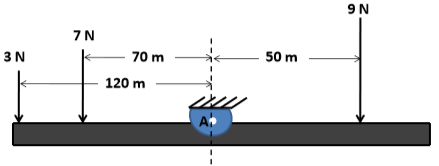Mining Engineering - (MN) 2018 2018 GATE Paper (Practice Test) - Question 55

The experiment to determine permeability of a soil sample is illustrated below. The crosssectional area of the sample is 20.0 cm2. The permeability of the soil sample in cm/s is ______________.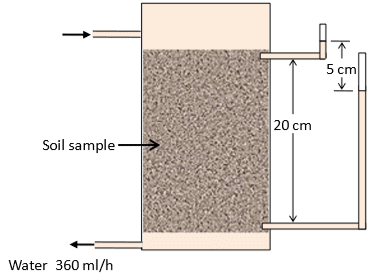Mining Engineering - (MN) 2018 2018 GATE Paper (Practice Test) - Question 56

In underground face blasting, the pull is found to be 10% less than the drillhole length. The headings of 3.6 m width are supported by prop density of 1.44/m2. The hole length is 1.5 m, and 6 rounds of blasting are done in the panel per shift. The number of props to be erected in a shift is ____________________.

Mining Engineering - (MN) 2018 2018 GATE Paper (Practice Test) - Question 57

The immediate roof of a mine is supported by bolts of length 1.5 m, arranged in 1.2 m x 1.2 m grid pattern. If the unit weight of roof rock is 2.25 tonne/m3 and load carrying capacity of each bolt is 7.5 tonne, the factor of safety of the support system is _______________.

Mining Engineering - (MN) 2018 2018 GATE Paper (Practice Test) - Question 58

In a level terrain, a vertical orebody of 20 m uniform width is worked by surface mining method. The density of the ore is 2.5 tonne/m3. The ultimate pit has a depth of 60 m, width of 20 m at pit bottom, and a pit slope of 45o. The overall stripping ratio for this condition in m3/tonne is ____________________.

Mining Engineering - (MN) 2018 2018 GATE Paper (Practice Test) - Question 59

A 20 m long steel tape used for survey is found to be short by 10 cm. If the area measured with the steel tape is 5000 m2, the actual area in m2 is __________________.

Mining Engineering - (MN) 2018 2018 GATE Paper (Practice Test) - Question 60

The following figure shows the designed blast pattern of a bench. The explosive column is charged at 18 kg/m. If the unit weight of the blasted material is 2.5 tonne/m3, the powder factor for the blast in tonne/kg is _______________.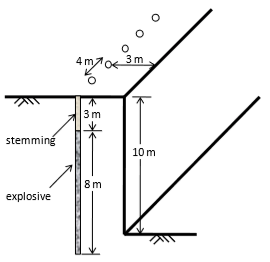Mining Engineering - (MN) 2018 2018 GATE Paper (Practice Test) - Question 61

Two points on the equator have longitudes 55oE and 25oW. Considering radius of earth as 6400 km, the distance between the two points in km is ________________.

Mining Engineering - (MN) 2018 2018 GATE Paper (Practice Test) - Question 62

Sum of the series 5, 10, 15, ……….., 500 is __________________.

Mining Engineering - (MN) 2018 2018 GATE Paper (Practice Test) - Question 63

The sample standard deviation for the following set of observations is _______________. 40, 45, 50 and 55

Mining Engineering - (MN) 2018 2018 GATE Paper (Practice Test) - Question 64

For the given function f ( x, y ) = ( 3 + x ) ( 4 + y ) , the value of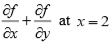and y= 1 is_____________________.

Mining Engineering - (MN) 2018 2018 GATE Paper (Practice Test) - Question 65

Given y = x 2 + x + 6 , the value of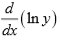at x = 2 is ________________.

## GATE Past Year Papers for Practice (All Branches)

407 docs|127 tests
Information about Mining Engineering - (MN) 2018 2018 GATE Paper (Practice Test) Page
In this test you can find the Exam questions for Mining Engineering - (MN) 2018 2018 GATE Paper (Practice Test) solved & explained in the simplest way possible. Besides giving Questions and answers for Mining Engineering - (MN) 2018 2018 GATE Paper (Practice Test), EduRev gives you an ample number of Online tests for practice

## GATE Past Year Papers for Practice (All Branches)

407 docs|127 tests